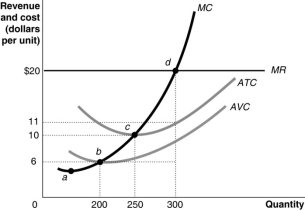• FAQ
• Contact/ Homework Answers / Economics / 31) The supply curve of a perfectly competitive firm in
Not my Question
Flag Content

# Question

31) The supply curve of a perfectly competitive firm in the short run is

A) the firm's average variable cost curve.

B) the portion of the firm's marginal cost curve below the minimum point of the average variable cost curve.

C) the portion of the firm's marginal cost curve above the minimum point of the average variable cost curve.

D) the portion of the firm's marginal cost curve above the minimum point of the average total cost curve.

32) A perfectly competitive firm's short-run supply curve is

A) upward sloping and is the portion of the marginal cost curve that lies above the average total cost curve.

B) upward sloping and is the portion of the marginal cost curve that lies above the average variable cost curve.

C) perfectly elastic at the market price.

D) horizontal at the minimum average total cost.

33) The minimum point on the average variable cost curve is called

A) the shutdown point.

B) the break-even point.

C) the loss minimizing point.

D) the point of diminishing returns.

34) If a perfectly competitive firm's total revenue is less than its total variable cost, the firm

A) should raise its price above its average variable cost.

B) should continue to produce and increase its demand.

C) should stop production by shutting down temporarily.

D) should adopt new technology in order to lower its costs of production.

35) In the mid-1990s, cattle ranchers in the United States kept raising cattle even though prices were at a ten-year low and below average total cost. What is the likely explanation for this?

A) Continuing to operate resulted in smaller losses than would have been incurred by shutting down.

B) The ranchers were hoping to receive government subsidies.

C) The exit costs were too high.

D) Cattle is an important source of protein and its production is essential for the United States.

Figure 12-1036) Refer to Figure 12-10.  The firm's short-run supply curve is its

A) marginal cost curve.

B) marginal cost curve from b and above.

C) marginal cost curve from c and above.

D) marginal cost curve from d and above.

37) Refer to Figure 12-10.  Total revenue at the profit-maximizing level of output is

A) \$1,200.

B) \$2,500.

C) \$4,800.

D) \$6,000.

38) Refer to Figure 12-10.  The total cost at the profit-maximizing output level equals

A) \$4,800.

B) \$3,300.

C) \$2,500.

D) \$1,800.

39) Refer to Figure 12-10.  At the profit-maximizing output level, the firm earns

A) zero economic profit.

B) a profit of \$600.

C) a profit of \$1,200.

D) a profit of \$2,700.

40) In the short run, a firm that incurs losses might choose to produce rather than shut down if the amount of its revenue is less than its fixed cost.

## Solution 5 (1 Ratings )

Solved
Economics 2 Months Ago 40 Views